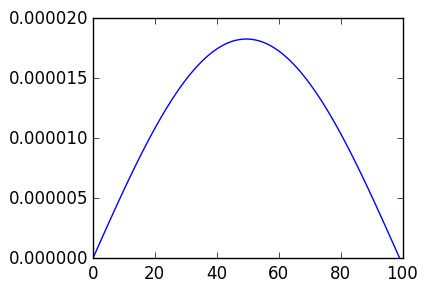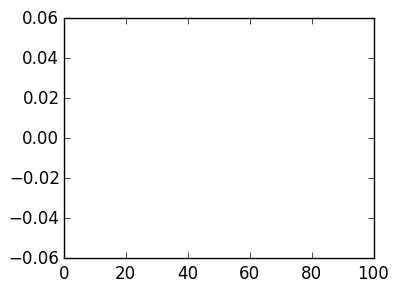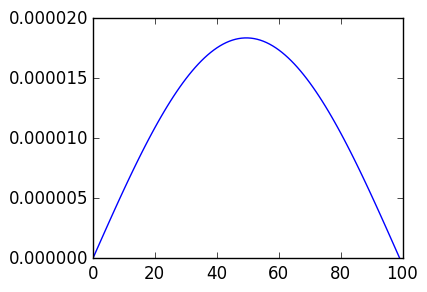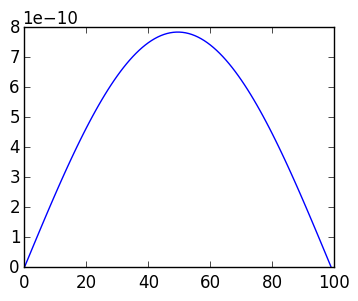# Lab 12: Time-evolution PDEs with boundary conditions¶

In lectures we saw that we can solve the heat equation with periodic boundary conditions:

$$u_t = u_{\theta\theta}, \qquad u(0,\theta) = u_0(\theta), \qquad u \hbox{ is periodic}$$

where initial condition is also periodic. This proceeded by either using either expm, ode45, or discretizing the forward Euler method in time

$$\mathbf w_{k+1} = \mathbf w_k + Δt D_n^2 \mathbf w_k$$

or the backward Euler method in time

$$\mathbf w_{k+1} = (I-Δt D_n^2)^{-1}\mathbf w_k$$

In this lecture, we will investigate the heat equation with Dirichlet boundary conditions:

$$u_t = u_{xx}, \qquad u(0,x) = u_0(x), u(t,0) = u(t,1) =0$$

where $u_0(x)$ also satisfies the Dircihlet conditions: $u_0(0) = u_0(1) = 0$.

## Failure of expm and ode45¶

Define the $n-1 \times n+1$ matrix

$$\tilde D_n^2 \triangleq D_{n-1} D_n = {1 \over h^2} \begin{pmatrix} 1 & -2 & 1 \cr & 1 & -2 & 1\cr &&\ddots & \ddots & \ddots \cr &&&1 & -2 & 1\end{pmatrix}$$

Question 1(a) Construct a function D2(h,n) that returns the $n-1 \times n+1$ matrix $\tilde D_n^2$, without checking the lecture notes.

In :
function D2(h,n)
ret=zeros(n-1,n+1)
for k=1:n-1
ret[k,k]=ret[k,k+2]=1/h^2
ret[k,k+1]=-2/h^2
end
ret
end

Out:
D2 (generic function with 1 method)

Question 1(b) Why is it not the case that

$$u(t) \approx \exp(\tilde D_n^2 t)$$

when we have Dirichlet boundary conditions? Try evaluating expm on D2(0.1,10) to confirm your explanation.

In :
expm(D2(0.1,10))

LoadError: DimensionMismatch("matrix is not square")

in chksquare at /Users/solver/Projects/julia/usr/lib/julia/sys.dylib
in expm! at linalg/dense.jl:193
in expm at linalg/dense.jl:186

Question 1(c) Why can't we solve the equation with ode45?

## Forward Euler method¶

We will now implement the forward Euler method. However, we need to modify it to incorporate boundary conditions.

Question 2(a) Why can't you implement the forward Euler scheme

$$\mathbf w_{k+1} = \mathbf w_k + Δt \tilde D_n^2 \mathbf w_k?$$

Question 2(b) Construct a forward Euler scheme that only modifies the interior points, that is the 2:end-1 entries of the vector, for $u_0(x) = x(1-x)\exp(x)$. Plot the solution at time $t=1$, where $n=99$, $\Delta x = 1/(n+1)$ and $\Delta t = \Delta x^2/2$.

In :
Δt=Δx^2/2

1/Δt

Out:
20000.0
In :
using PyPlot

n=99

Δx=1/(n+1)
Δt=Δx^2/2

x=linspace(0.,1.,n+1)  # Grid points

K=20000  # number of time steps to get to T=1
u0=x->x.*(1-x).*exp(x)  # initial condition

A=D2(Δx,n)

w=Array(Vector{Float64},K+1)
w=u0(x)

for k=1:K
w[k+1]=w[k]
w[k+1][2:end-1]+=Δt*A*w[k]
end

plot(w[K+1])Out:
1-element Array{Any,1}:
PyObject <matplotlib.lines.Line2D object at 0x3153892d0>

Question 2(c) What goes wrong if $\Delta t = \Delta x^2$?

In :
n=99

Δx=1/(n+1)
Δt=Δx^2

x=linspace(0.,1.,n+1)  # Grid points

K=20000  # number of time steps to get to T=1
u0=x->x.*(1-x).*exp(x)  # initial condition

A=D2(Δx,n)

w=Array(Vector{Float64},K+1)
w=u0(x)

for k=1:K
w[k+1]=w[k]
w[k+1][2:end-1]+=Δt*A*w[k]
end

plot(w[K+1])Out:
1-element Array{Any,1}:
PyObject <matplotlib.lines.Line2D object at 0x315707f90>

# Backward Euler and boundary conditions¶

We will now show that backward Euler easily allows for boundary conditions. But we need to put in some thought into how boundary conditions are incorporated:

Question 3(a) Why does the following backward Euler construction break down: $$\mathbf w_{k+1} = (I-Δt \tilde D_n^2)^{-1}\mathbf w_k$$

Question 3(b) How would you incorporate the boundary conditions in the linear solve? Repeat 2(b) with the backward Euler method.

In :
n=99

Δx=1/(n+1)
Δt=Δx^2/2

x=linspace(0.,1.,n+1)  # Grid points

K=20000  # number of time steps to get to T=1
u0=x->x.*(1-x).*exp(x)  # initial condition

L=D2(Δx,n)
B0=[1 zeros(1,n)]
B1=[zeros(1,n) 1]
M=[B0; eye(n+1)[2:end-1,:]-Δt*L; B1]

w=Array(Vector{Float64},K+1)
w=u0(x)

for k=1:K
w[k+1]=M\w[k]
end

plot(w[K+1])Out:
1-element Array{Any,1}:
PyObject <matplotlib.lines.Line2D object at 0x315c2ca10>

Question 3(c) Do you see the same issues as in forward Euler in 2(c) when using $\Delta t =\Delta x^2$ in backward Euler?

In :
n=99

Δx=1/(n+1)
Δt=Δx^2

x=linspace(0.,1.,n+1)  # Grid points

K=20000  # number of time steps to get to T=1
u0=x->x.*(1-x).*exp(x)  # initial condition

L=D2(Δx,n)
B0=[1 zeros(1,n)]
B1=[zeros(1,n) 1]
M=[B0; eye(n+1)[2:end-1,:]-Δt*L; B1]

w=Array(Vector{Float64},K+1)
w=u0(x)

for k=1:K
w[k+1]=M\w[k]
end

plot(w[K+1])Out:
1-element Array{Any,1}:
PyObject <matplotlib.lines.Line2D object at 0x315f50d90>